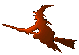Hex  1.0 Hydrogen-electron collision solver
DenseMatrix< T > Class Template Reference

#include <matrix.h>

## Public Member Functions

DenseMatrix ()

DenseMatrix (int rows, int cols)

DenseMatrix (int rows, int cols, const ArrayView< T > data)

ArrayView< T > data ()

const ArrayView< T > data () const

size_t size () const

int cols () const

int rows () const

T * begin ()

T const * begin () const

## Detailed Description

### template<class T> class DenseMatrix< T >

Base class for row- and column- oriented matrices. The data are stored in a one-dimensional array. The class has a basic data access interface; however, the main purpose is that it is the base class of the storage-order-specialized classes RowMatrix and ColMatrix. It is recommended to use the latter two when working with dense matrices.

## Constructor & Destructor Documentation

template<class T>
 DenseMatrix< T >::DenseMatrix ( )
inline
template<class T>
 DenseMatrix< T >::DenseMatrix ( int rows, int cols )
inline
template<class T>
 DenseMatrix< T >::DenseMatrix ( int rows, int cols, const ArrayView< T > data )
inline

## Member Function Documentation

template<class T>
 T* DenseMatrix< T >::begin ( )
inline
template<class T>
 T const* DenseMatrix< T >::begin ( ) const
inline
template<class T>
 int DenseMatrix< T >::cols ( ) const
inline
template<class T>
 ArrayView DenseMatrix< T >::data ( )
inline
template<class T>
 const ArrayView DenseMatrix< T >::data ( ) const
inline
template<class T>
 int DenseMatrix< T >::rows ( ) const
inline
template<class T>
 size_t DenseMatrix< T >::size ( ) const
inline

The documentation for this class was generated from the following file: